## How We Did Vibrations

The C++ program, written by Andrew Schultz, used to create the .xyz animated files of the vibrations is available here. chem.h and vector.h are also available.

### Setup

The program first reads in the file to obtain the coordinates and element of each atom. The next thing the program does is to allow for the molecule to be enlarged or shrunk. Because the .xyz file format does not include bonds, Chime and Rasmol must decide where the bonds are, based on inter-atomic distances. Because vibration cause the atoms to be closer or farther apart, bonds can be broken and unwanted bonds can be formed. The vibration program prevents this by allowing the molecule to be scaled up or down. Here is a water molecule being bent more than in the water page, which illustrates this possibility:

At this point the program calculates the center of mass of the molecule. Next the program asks for how many frames should be in the .xyz file. Chime plays the file forwards and then backwards, so only half a loop's worth of frames are needed.

The program asks for the percent the bonds should be stretched in symmetric and asymmetric vibrations and then how many degrees the bond should bend.

### Symmetric and Asymmetric Vibrating

The program assumes that the first atom is the central atom and that the second two are bonded to it. The two bond lengths undergo simple harmonic motion, centered about the original bond distance. If it is symmetric vibration, the bonds vibrate in phase, while in asymmetric vibration, they vibrate out 180 degrees out of phase. After new atomic positions are calculated, a center of mass correction is done. Then a rotational motion correction is done. Then the coordinates are output to a file and the loop continues with the next frame.

### Bending

In bending, the bond angle undergoes simple harmonic motion. To do this, a basis must first be found for the system. The program is not capable of calculating a basis for a linear molecule, so if the molecule is linear, a minuscule shift in the coordinates of one of the atoms will allow the program without effecting the final display. The vector from the center atom to the second atom and then normalized is taken as the first basis vector. If the coordinates of atom i are ci,The Gram-Schmidt process is used to find the second basis vector: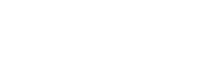Using this new basis, the angle of the first bond is 0 and the angle of the second bond is: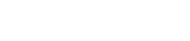Next each of these angles undergoes simple harmonic motion. If at a particular time, the angle of a bond is phi,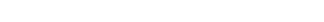A center of mass correction and then a rotational motion correction is applied to these new coordinates. Then the coordinates are written to the file and the loop continues with the next frame.

### Center of Mass Correction

To correct for any translation motion caused by the change in atomic positions, the center of mass with the new positions is calculated. Then the change in the center of mass is subtracted from every atomic coordinate.

### Rotational Motion Correction

Although rotational motion caused by the vibrations was not a significant problem in the molecules we have used as examples, it's potential to cause problems definitely exists.
The first step is to approximate the angular momentum and moment of inertia of the molecule.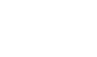These values are approximate because the radius is not necessarily constant during this proccess. The angular velocity is calculated from this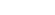Next the atomic coordinates are corrected by subtracting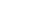from each of them.

### HTML

This section assumes a basic knowledge of
HTML.
In the HTML implementation of vibrating molecules, we have used the following syntax:
```<embed src="filename.xyz" display3d="ball&stick" width="250" height="250"
animfps="10" startanim="true" script="zoom 200">
```
The width and height parameters specify the size of the box used to display the molecule. The animfps parameter sets the number of frames per second. The startanim sets the animation starting once the molecule loads.